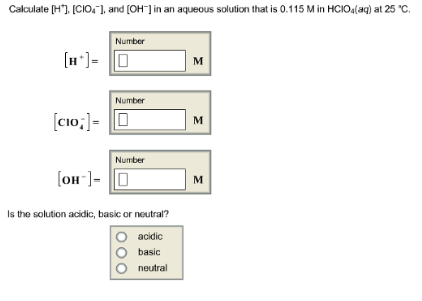# Problem: Calculate [H+], and [OH-] in an aqueous solution that is 0.115 M in HCLO 4(aq) at 25°C. Is the solution acidic, basic or neutral?

###### FREE Expert Solution
93% (35 ratings)###### Problem Details

Calculate [H+], and [OH-] in an aqueous solution that is 0.115 M in HCLO 4(aq) at 25°C.

Is the solution acidic, basic or neutral?